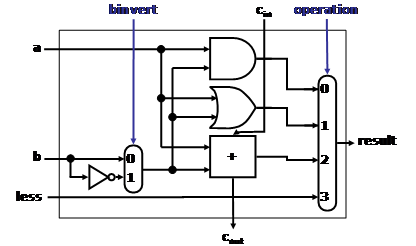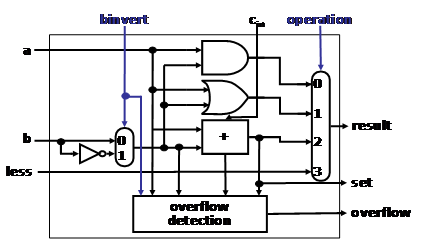Computer Organization and Structure

Homework #3

Due: 2008/11/4

1.      The ALU supported set on less than (slt) using just the sign bit of the adder. Let’s try a set on less than operation using the values -7ten and 6ten. To make it simpler to follow the example, let’s limit the binary representations to 4 bits: 1001two and 0110two.

1001two – 0110two = 1001two + 1010two = 0011two

This result would suggest that -7ten > 6ten, which is clearly wrong. Hence we must factor in overflow in the decision. Modify the 1-bit ALU in the following figures to handle slt correctly.Figure 1: A 1-bit ALU that performs AND, OR, and addition on a and b or b’.Figure 2: A 1-bit ALU for the most significant bit.

2.      Given the bit pattern:

1010 1101 0001 0000 0000 0000 0000 0010

what does it represent, assuming that it is

a.         a two’s complement integer?

b.        an unsigned integer?

c.         a single precision floating-point number?

d.        a MIPS instruction?

3.      Add 2.85ten x 103 to 9.84ten x 104 and add 3.63ten x 104 to 6.87ten x 103, respectively, assuming that you have only three significant digits, first with guard and round digits and then without them.

4.      Consider two different implementations, I1 and I2, of the same instruction set. There are three classes of instructions (A, B, and C) in the instruction set. I1 has a clock rate of 6GHz, and I2 has a clock rate of 3GHz. The average number of cycles for each instruction class on I1 and I2 is given in the following table:

 Class CPI on M1 CPI on M2 C1 Usage C2 Usage C3 Usage A 2 1 40% 40% 50% B 3 2 40% 20% 25% C 5 2 20% 40% 25%

The table also contains a summary of average proportion of instruction classes generated by three different compilers. C1 is a compiler produced by the makers of I1, C2 is produced by the makers of I2, and the other compiler is a third-party product. Assume that each compiler uses the same number of instructions for a given program but that the instruction mix is as described in the above table. Using C1 on both I1 and I2, how much faster can the makers of I1 claim I1 is compared to I2? Using C2, how much faster can the makers of I2 claim that I2 is compared to I1? If you purchase I1, which compiler would you use? If you purchased I2, which compiler would you use? Which computer and compiler would you purchase if all other criteria are identical, including cost?